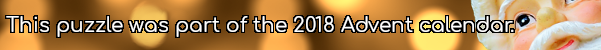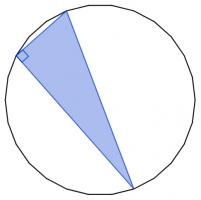mscroggs.co.uk
mscroggs.co.uksubscribe

# Puzzles

## 19 DecemberThe diagram below shows three squares and five circles. The four smaller circles are all the same size, and the red square's vertices are the centres of these circles.
The area of the blue square is 14 units. What is the area of the red square?

## 5 December28 points are spaced equally around the circumference of a circle. There are 3276 ways to pick three of these points. The three picked points can be connected to form a triangle. Today's number is the number of these triangles that are isosceles.

## 2 DecemberYou have 15 sticks of length 1cm, 2cm, ..., 15cm (one of each length). How many triangles can you make by picking three sticks and joining their ends?
Note: Three sticks (eg 1, 2 and 3) lying on top of each other does not count as a triangle.
Note: Rotations and reflections are counted as the same triangle.

## 23 DecemberToday's number is the area of the largest area rectangle with perimeter 46 and whose sides are all integer length.

## 12 DecemberThere are 2600 different ways to pick three vertices of a regular 26-sided shape. Sometime the three vertices you pick form a right angled triangle.These three vertices form a right angled triangle.
Today's number is the number of different ways to pick three vertices of a regular 26-sided shape so that the three vertices make a right angled triangle.

## Equal lengths

The picture below shows two copies of the same rectangle with red and blue lines. The blue line visits the midpoint of the opposite side. The lengths shown in red and blue are of equal length.
What is the ratio of the sides of the rectangle?

## Is it equilateral?

In the diagram below, $$ABDC$$ is a square. Angles $$ACE$$ and $$BDE$$ are both 75°.
Is triangle $$ABE$$ equilateral? Why/why not?

## Bending a straw

Two points along a drinking straw are picked at random. The straw is then bent at these points. What is the probability that the two ends meet up to make a triangle?

## Archive

Show me a random puzzle
▼ show ▼